Patchwork Punk
August 9, 2016

# Patchwork Punk

VIEW ALL PHOTOS VIEW SLIDESHOW
GET THE HOW-TO• Formula A

• Formula B

90g Bed Head by TIGI® Colour Trip Violet + 15g Blue

• Formula C

40g Clear + 3g Green

• Formula D

15g Pale Violet + 15g Clear + 2g Orange + 1g Pink# Patchwork Punk

Push the boundaries of color with the new Bed Head Colour Trip semi-permanent line from TIGI®!  The overall effect of this look is pastel, but with strong focal points on each side of the head. How far will you go?

Colorist: Christel Lundqvist
Manufacturer: TIGI, @tigiprofessionals

## COLOR FORMULAS• Formula A

• Formula B

90g Bed Head by TIGI® Colour Trip Violet + 15g Blue

• Formula C

40g Clear + 3g Green

• Formula D

15g Pale Violet + 15g Clear + 2g Orange + 1g Pink

## HOW-TO STEPS•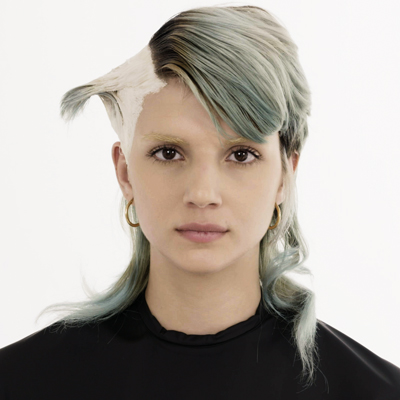• Formula A

• Formula B

90g Bed Head by TIGI® Colour Trip Violet + 15g Blue

• Formula C

40g Clear + 3g Green

• Formula D

15g Pale Violet + 15g Clear + 2g Orange + 1g Pink

1

To ensure vibrancy, prelighten the side panel with Formula A, lifting the hair to a pale yellow undertone.

•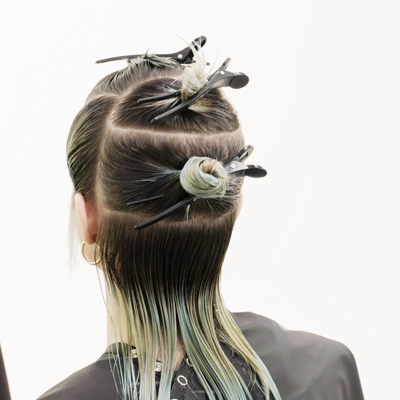• Formula A

• Formula B

90g Bed Head by TIGI® Colour Trip Violet + 15g Blue

• Formula C

40g Clear + 3g Green

• Formula D

15g Pale Violet + 15g Clear + 2g Orange + 1g Pink

2

Begin by taking a radial parting, then subdivide the back into three sections.

•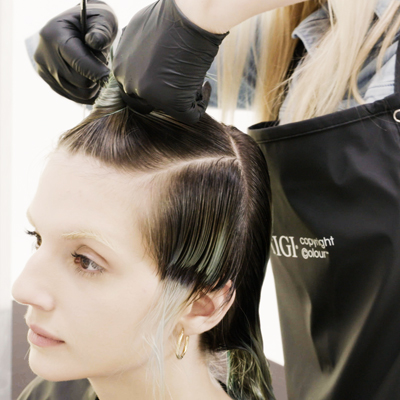• Formula A

• Formula B

90g Bed Head by TIGI® Colour Trip Violet + 15g Blue

• Formula C

40g Clear + 3g Green

• Formula D

15g Pale Violet + 15g Clear + 2g Orange + 1g Pink

3

From the radial parting, take a rectangular section to the forehead. This will give you a total of six sections.

•• Formula A

• Formula B

90g Bed Head by TIGI® Colour Trip Violet + 15g Blue

• Formula C

40g Clear + 3g Green

• Formula D

15g Pale Violet + 15g Clear + 2g Orange + 1g Pink

4

Take vertical sections at the nape area and apply Formula B to the root. With a freehand blending technique, apply Formula C through the midlengths and ends.

•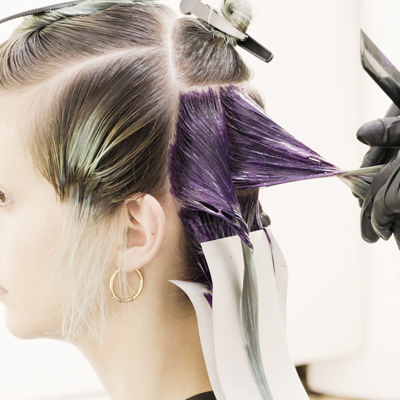• Formula A

• Formula B

90g Bed Head by TIGI® Colour Trip Violet + 15g Blue

• Formula C

40g Clear + 3g Green

• Formula D

15g Pale Violet + 15g Clear + 2g Orange + 1g Pink

5

Progressing onto the next section, take vertical sections and apply Formula B to the root area, then twist the section 180 degrees and apply Formula C through the ends.

•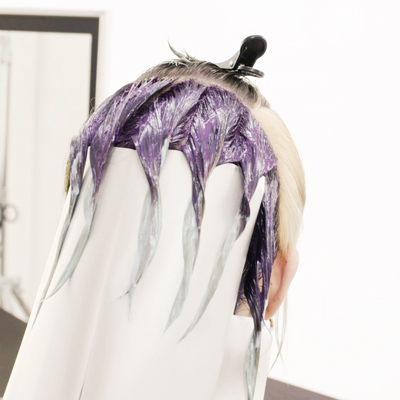• Formula A

• Formula B

90g Bed Head by TIGI® Colour Trip Violet + 15g Blue

• Formula C

40g Clear + 3g Green

• Formula D

15g Pale Violet + 15g Clear + 2g Orange + 1g Pink

6

Continue working in the same way until the entire back section is complete.

•• Formula A

• Formula B

90g Bed Head by TIGI® Colour Trip Violet + 15g Blue

• Formula C

40g Clear + 3g Green

• Formula D

15g Pale Violet + 15g Clear + 2g Orange + 1g Pink

7

Proceed onto the side section. Apply Formula B from the roots straight through to the midlengths and ends.

•• Formula A

• Formula B

90g Bed Head by TIGI® Colour Trip Violet + 15g Blue

• Formula C

40g Clear + 3g Green

• Formula D

15g Pale Violet + 15g Clear + 2g Orange + 1g Pink

8

Repeat the application on the opposite side using Formula D.

•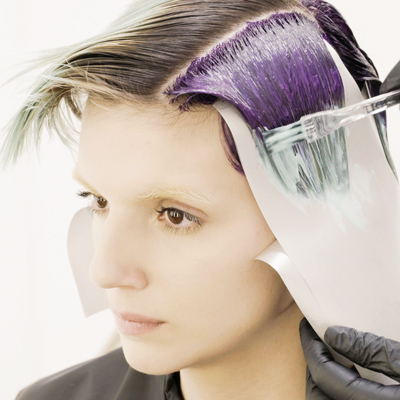• Formula A

• Formula B

90g Bed Head by TIGI® Colour Trip Violet + 15g Blue

• Formula C

40g Clear + 3g Green

• Formula D

15g Pale Violet + 15g Clear + 2g Orange + 1g Pink

9

Proceed to the remaining top section and apply Formula B to the roots. With a freehand blending technique, apply Formula C to the ends. Process for up to 20 minutes. Emulsify, rinse and remove using the Bed Head Colour Goddess range.

•• Formula A

• Formula B

90g Bed Head by TIGI® Colour Trip Violet + 15g Blue

• Formula C

40g Clear + 3g Green

• Formula D

15g Pale Violet + 15g Clear + 2g Orange + 1g Pink

10